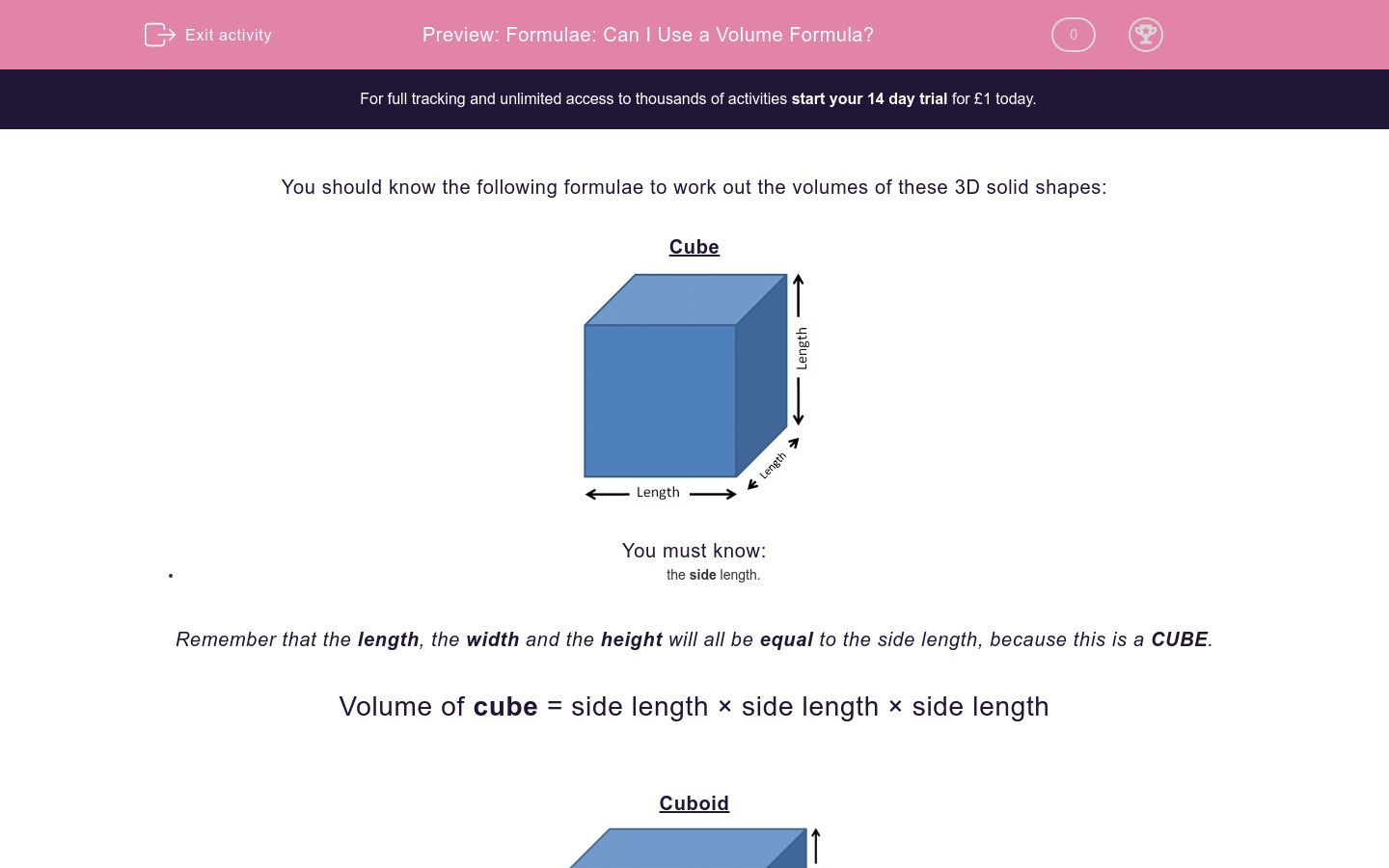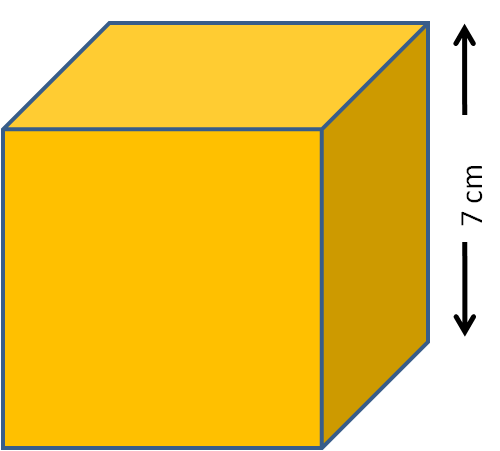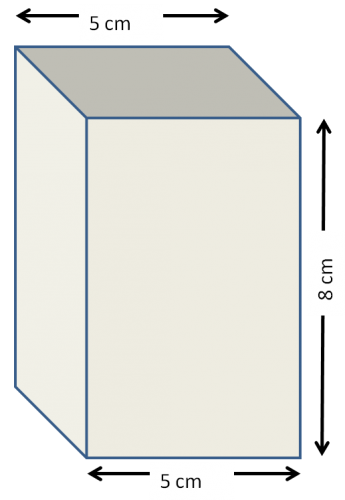# Formulae: Can I Use a Volume Formula?

In this worksheet, students must recognise when it is possible to use formulae for the volume of the given shapes.Key stage:  KS 2

Curriculum topic:   Maths and Numerical Reasoning

Curriculum subtopic:   3D Shapes

Difficulty level:### QUESTION 1 of 10

You should know the following formulae to work out the volumes of these 3D solid shapes:

CubeYou must know:

• the side length.

Remember that the length, the width and the height will all be equal to the side length, because this is a CUBE.

Volume of cube = side length × side length × side length

CuboidYou must know:

• the length
• the width
• the height.

Volume of cuboid = length × width × height

Example 1

Can a volume formula be used to work out the volume of this cuboid, and if so, which one is best?This is a cuboid.

We must know:

• the length
• the width
• the height.

The length is 15 cm, the width is 4 cm and the height is 8 cm.

Yes, we can use the volume formula:

Volume of cuboid  = length × width × height

Example 2

Can a volume formula be used to work out the volume of this cube, and if so, which one is best?This is a cube.

We must know:

• the side length.

The side length is 7 cm, so the width and the height are also 7 cm.

Yes, we can use the volume formula:

Volume of cube  = side length × side length × side length

Example 3

Can a volume formula be used to work out the volume of this cuboid, and if so, which one is best?This is a cuboid.

We must know:

• the length
• the width
• the height.

The length is 9 cm, the width is 4 cm but we do not know the height.

No, we cannot use a volume formula.

NB The length, the width and the height are all interchangeable with each other, depending on how you look at a cuboid.

Choose the best volume formula to be used to work out the volume of this cuboid.volume = side length × side length × side length

volume = length × width × height

not possible to work out volume

Choose the best volume formula to be used to work out the volume of this cube.volume = side length × side length × side length

volume = length × width × height

not possible to work out volume

Choose the best volume formula to be used to work out the volume of this cuboid.volume = side length × side length × side length

volume = length × width × height

not possible to work out volume

Choose the best volume formula to be used to work out the volume of this cuboid.volume = side length × side length × side length

volume = length × width × height

not possible to work out volume

Choose the best volume formula to be used to work out the volume of this cube.volume = side length × side length × side length

volume = length × width × height

not possible to work out volume

Choose the best volume formula to be used to work out the volume of this cuboid.volume = side length × side length × side length

volume = length × width × height

not possible to work out volume

Choose the best volume formula to be used to work out the volume of this cube.volume = side length × side length × side length

volume = length × width × height

not possible to work out volume

Choose the best volume formula to be used to work out the volume of this cuboid.volume = side length × side length × side length

volume = length × width × height

not possible to work out volume

Choose the best volume formula to be used to work out the volume of this cube.volume = side length × side length × side length

volume = length × width × height

not possible to work out volume

Choose the best volume formula to be used to work out the volume of this cuboid, which has a square end as shown.volume = side length × side length × side length

volume = length × width × height

not possible to work out volume

• Question 1

Choose the best volume formula to be used to work out the volume of this cuboid.volume = length × width × height
EDDIE SAYS
This is a cuboid.
We know the length, the width and the height.
• Question 2

Choose the best volume formula to be used to work out the volume of this cube.volume = side length × side length × side length
EDDIE SAYS
This is a cube.
All the side lengths are 5 cm.
• Question 3

Choose the best volume formula to be used to work out the volume of this cuboid.not possible to work out volume
EDDIE SAYS
This is a cuboid.
We know two of the length, the width and the height, but we need all three.
• Question 4

Choose the best volume formula to be used to work out the volume of this cuboid.not possible to work out volume
EDDIE SAYS
This is a cuboid.
The 5 cm is shown twice as the width.
We know two of the length, the width and the height, but we need all three.
• Question 5

Choose the best volume formula to be used to work out the volume of this cube.volume = side length × side length × side length
EDDIE SAYS
This is a cube.
All the side lengths are 5 mm.
• Question 6

Choose the best volume formula to be used to work out the volume of this cuboid.volume = length × width × height
EDDIE SAYS
This is a cuboid.
The 5 cm is shown twice as the width.
We know the length, the width and the height, so we can use the volume formula for a cuboid.
• Question 7

Choose the best volume formula to be used to work out the volume of this cube.volume = side length × side length × side length
EDDIE SAYS
This is a cube.
All the side lengths are 9 cm even though only two of them are shown.
• Question 8

Choose the best volume formula to be used to work out the volume of this cuboid.not possible to work out volume
EDDIE SAYS
This is a cuboid.
The 15 cm is shown three times as the length.
We do not know the width or the height.
• Question 9

Choose the best volume formula to be used to work out the volume of this cube.not possible to work out volume
EDDIE SAYS
This is a cube.
All the side lengths are the same but we have no information on these lengths.
• Question 10

Choose the best volume formula to be used to work out the volume of this cuboid, which has a square end as shown.volume = length × width × height
EDDIE SAYS
This is a cuboid.
The length is 20 cm.
The width is 9 cm as shown.
The height is also 9 cm because the end is a square.
---- OR ----

Sign up for a £1 trial so you can track and measure your child's progress on this activity.

### What is EdPlace?

We're your National Curriculum aligned online education content provider helping each child succeed in English, maths and science from year 1 to GCSE. With an EdPlace account you’ll be able to track and measure progress, helping each child achieve their best. We build confidence and attainment by personalising each child’s learning at a level that suits them.

Get started